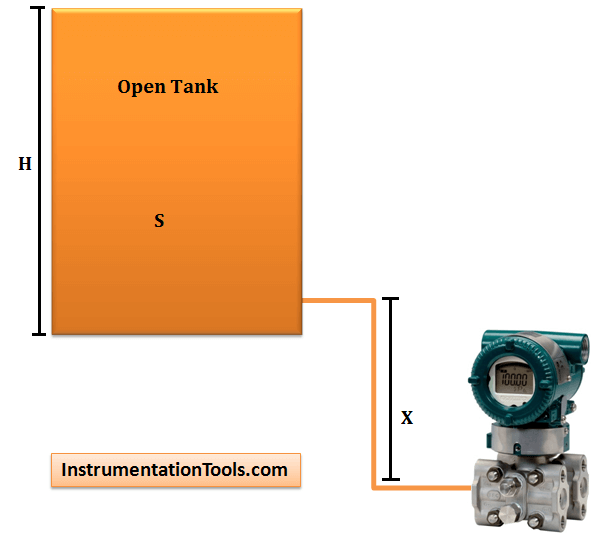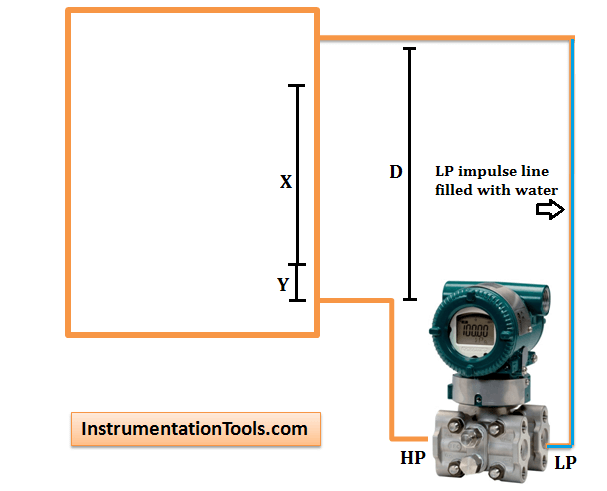Home » Zero Suppression and Zero Elevation Calculations

# Zero Suppression and Zero Elevation Calculations

This article provides information on Zero Suppression and Zero Elevation Calculations used in differential pressure level transmitter.

### What is Zero Suppression ?

In some cases, the level transmitter has to be mounted X meters below the base of an open tank as shown in below figure.The liquid in the high pressure impulse line exerts a constant pressure (P = S . X) on the high pressure side.

That is, the pressure on the high pressure side of the DP Transmitter is always higher than the actual pressure exerted by the liquid column in the tank by (SG . X) – so the reading will be in error high.

This constant pressure would cause an output signal that is higher than 4 mA when the tank is empty and above 20 mA when it is full.

When the liquid level is at H meters, pressure on the high pressure side of the transmitter will be:

#### ΔP = Phigh – Plow = S . H + S . X

The transmitter has to be negatively biased by a value of S.X so that the output of the transmitter is proportional to the tank level (S . H) only.

The above procedure is called Zero Suppression and it can be done during calibration of the transmitter.

### What is Zero Elevation ?

When a wet leg installation is used,the low pressure side of the level transmitter will always experience a higher hydrostatic pressure than the high pressure side.

This is due to the fact that the height of the wet leg (X) is always just greater than the maximum height of the liquid column (H) inside the tank.When the liquid level is at H meters, we have:

#### ΔP = Phigh – Plow = S . H – S . X = -S(X . H)

The differential pressure ΔP sensed by the transmitter is always a negative value (i.e. the low pressure side has a higher pressure than high pressure side).

To properly calibrate the transmitter, a positive bias (S . X) is needed to elevate the transmitter output.

This positive biasing technique is called zero elevation.

### Zero suppression calculationSpan = (x) (GL)

HW at minimum level = (z) (GS) + (y) (GL)

HW at maximum level = (z) (GS) + (x + y) (GL)

Where
GL = Specific gravity of tank liquid
GS = Specific gravity of seal liquid
HW = Equivalent head of water

x, y, and z as shown in above figure

#### Example:

Open tank with x = 80 inches

y = 5 inches

z = 10 inches
GL = 0.8
GS = 0.9

Span = (80)(0.8) = 64 inches

HW at minimum level = (10)(0.9) + (5)(0.8) = 13 inches

HW at maximum level = (10)(0.9) + (5 + 80)(0.8) = 77 inches

Calibrated Range = 13 to 77 inches head of water

### Zero elevation calculation Closed Tank With Wet LegSpan = (x)(GL) Wet Leg

HW at minimum level = (y)(GL) – (d)(GS)

HW at maximum level = (x + y)(GL) – (d)(GS)

Where
GL = Specific gravity of tank liquid
GS = Specific gravity of seal liquid
HW = Equivalent head of water

#### Example:

Closed tank with x = 70 inches y = 20 inches, and d = 100 inches,  GL = 0.8 Seal Liquid,  GS = 0.9

Span = (70)(0.8) = 56 inches

HW at minimum level = (20)(0.8) – (100)(0.9) = -74 inches

HW at maximum level = (70 + 20)(0.8) – (100)(0.9) = -18 inches

Calibrated Range = -74 to -18 inches head of water

(Minus signs indicate that the higher pressure is applied to the low pressure side of the transmitter.)

#### Articles You May Like :

Level Transmitter Compensation

Field Instrumentation Questions

DP Type Level Measurement

Fork Level Switches Principle

Remote mount Level Transmitter

#### 4-20mA Graphical CalculationsMay 6, 2016 at 1:35 am

. I am very thankful to you sir. Thanks for explaining about Zero Suppression and Zero Elevation.June 21, 2016 at 11:27 am

How about explaining how to calculate zero suppression and zero elevation when the level transmitter is above a closed tank and the upper head space is pressurized at 30 psig with air? This is one of the common uses for remote water level monitoring of the seal pots/tanks for the process pump seals.July 27, 2016 at 3:05 pm

Thanks sirAugust 14, 2016 at 11:58 am

Thank you Sir for all your best Information to sharing usDecember 22, 2016 at 6:32 am

Thanks you sirMarch 20, 2017 at 4:24 pm

Why we used upperline high in drum level or dearetor levelApril 19, 2017 at 6:50 pm

Thannks for refreshing the theory

This website uses cookies to improve your experience. We'll assume you're ok with this, but you can opt-out if you wish. Accept Read More

WordPress Image Lightbox

Send this to a friend